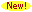Lecture Notes

Which linear fractional transformations induce rotations of the sphere? Notes to supplement the material on linear fractional maps presented in a first year graduate course. You find here a proof that a LFT induces, through stereographic projection, a rotation of the Riemann sphere if and only if it can be represented by a unitary (two by two) coefficient matrix.

Notes on the numerical range. These are lecture notes that set out the basic properties of the numerical range of a Hilbert space operator, most notably the Toeplitz-Hausdorff Theorem which shows that the numerical range is always a convex set. Along the way, the numerical ranges of two by two matrices are characterized. A proof is given of Hildebrandt's theorem, which states that if you intersect the closures of the numerical ranges of all operators similar to an operator T, what results is the closed convex hull of the spectrum of T.

Notes on the dynamics of linear operators  These are notes intended to complement lectures I gave in June 2001 at the Universities of Rome, Florence, and Padua in Italy. They discuss the notion of hypercyclicity for linear operators (some orbit is dense), paying particular attention to its origins in non-linear dynamics, and its connections with analytic function theory and functional analysis.

The Arzela-Ascoli Theorem. Notes to supplement the discussion of normal families and the Riemann Mapping Theorem in a first-year graduate course in complex variables

Notes on connectivity. Supplementary notes for a first-year graduate course in complex variables. The notes develop basic facts about connectivity and components in metric spaces, with emphasis on Euclidean space.

Notes on Differentiation. Supplementary notes for a first-year graduate course in complex analysis. Quick review of real differentiation in several variables, Conformality of stereographic projection, application to Mercator map projection.

A Gentle Introduction to Composition Operators. Lecture notes for the first half of a course I gave at the University of Padua (Italy) in June 1998.

Nonmeasurable sets and paradoxical decompositions. Supplementary notes for a first-year graduate course in real analysis.

Cyclic decomposition of a nilpotent matrix. Supplementary notes for the discusssion of Jordan Canonical form in a senior-level course in linear algebra.

Quadratic extension fields and geometric impossibilities. Supplementary notes for a first course in abstract algebra at the junior-senior level.Cyclic inner functions in Bergman spaces. An exposition of the ``Korenblum-Roberts Theorem'' characterizing inner functions that are cyclic for the forward shift on Bergman spaces.